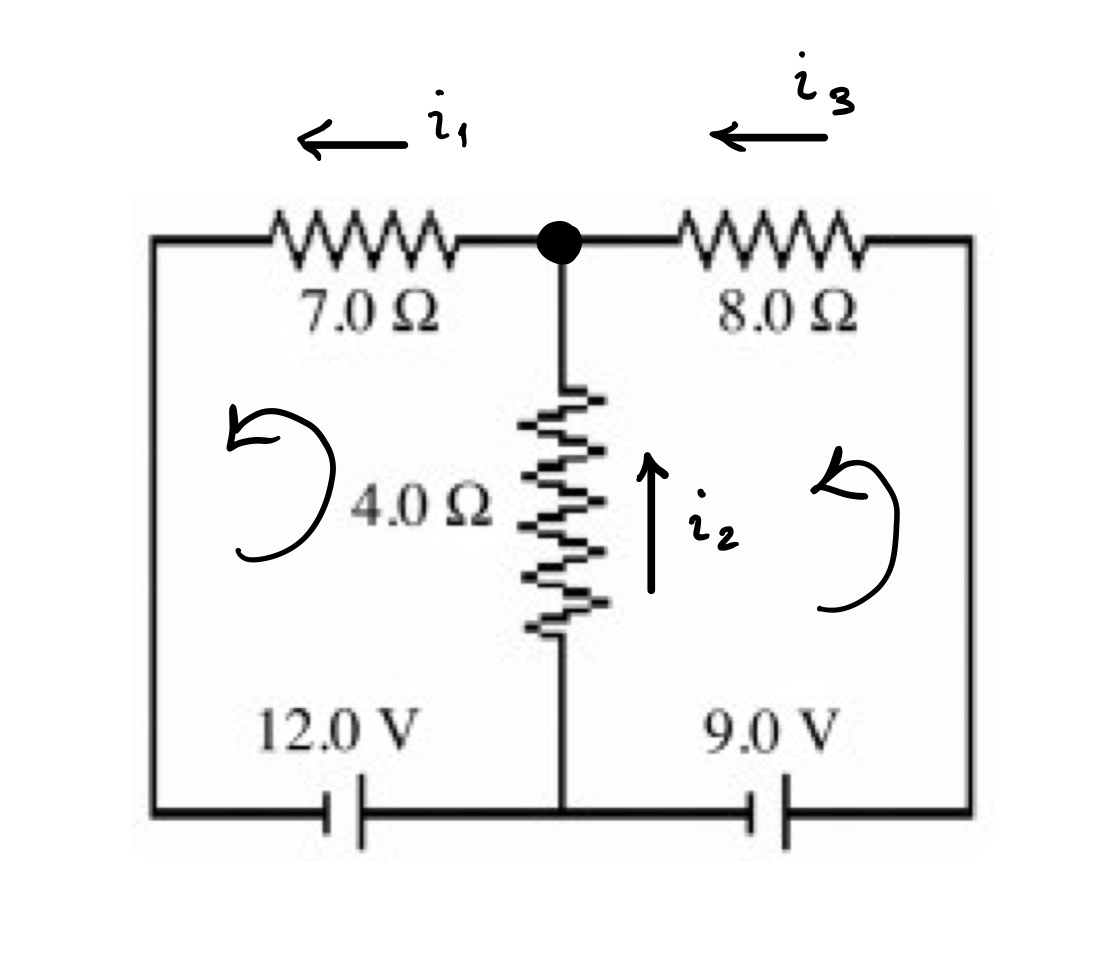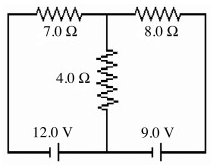# Problem: A) For the circuit shown in the figure, determine the current in the 7.0-Ω resistor.B) For the circuit shown in the figure, determine the current in the 8.0-Ω  resistor.C) For the circuit shown in the figure, determine the current in the 4.0-Ω  resistor.Please show detailed work.

###### FREE Expert Solution

Kirchhoff's loop rule:

$\overline{){\mathbf{\Sigma }}{\mathbf{V}}{\mathbf{=}}{\mathbf{0}}}$

A)Consider Kirchhoff's loop rule in the left loop.

We're assuming the current is moving in a clockwise direction in this loop.

The current is moving from the left to right through resistor 7.0Ω and top to bottom through resistor 4.0Ω.

Now we have:

$\begin{array}{rcl}\mathbf{-}{\mathbf{i}}_{\mathbf{1}}\mathbf{7}\mathbf{.}\mathbf{0}\mathbf{-}\mathbf{\left(}{\mathbf{i}}_{\mathbf{1}}\mathbf{-}{\mathbf{i}}_{\mathbf{2}}\mathbf{\right)}\mathbf{4}\mathbf{.}\mathbf{0}\mathbf{-}\mathbf{12}\mathbf{.}\mathbf{0}& \mathbf{=}& \mathbf{0}\\ \begin{array}{rc}\mathbf{-}{\mathbf{i}}_{\mathbf{1}}\mathbf{7}\mathbf{.}\mathbf{0}\mathbf{-}{\mathbf{i}}_{\mathbf{1}}\mathbf{4}\mathbf{.}\mathbf{0}\mathbf{+}{\mathbf{i}}_{\mathbf{2}}& \mathbf{4}\mathbf{.}\mathbf{0}\mathbf{-}\mathbf{12}\mathbf{.}\mathbf{0}\end{array}& \mathbf{=}& \mathbf{0}\\ \mathbf{-}\mathbf{11}\mathbf{.}\mathbf{0}{\mathbf{i}}_{\mathbf{1}}\mathbf{+}\mathbf{4}\mathbf{.}\mathbf{0}{\mathbf{i}}_{\mathbf{2}}\mathbf{-}\mathbf{12}\mathbf{.}\mathbf{0}& \mathbf{=}& \mathbf{0}\end{array}$

96% (485 ratings)###### Problem DetailsA) For the circuit shown in the figure, determine the current in the 7.0-Ω resistor.

B) For the circuit shown in the figure, determine the current in the 8.0-Ω  resistor.

C) For the circuit shown in the figure, determine the current in the 4.0-Ω  resistor.